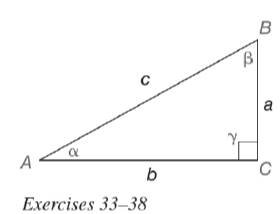Chapter 11.3, Problem 35EElementary Geometry For College St...

7th Edition
Alexander + 2 others
ISBN: 9781337614085

Solutions

Chapter
SectionElementary Geometry For College St...

7th Edition
Alexander + 2 others
ISBN: 9781337614085
Textbook Problem

In Exercises 33 to 38, we expand the list of trigonometric identities. As you may recall, an identity is a statement that is true for all permissible choices of the variable (see page 510.)a) For α ≠ 90 ∘ , prove the identity sec α = 1 cos α . b) Use your calculator to determine sec 82 ∘ .To determine

(a)

To prove:

For α90, the identity secα=1cosα.

Explanation

Given:

For α90, secα=1cosα.

Definition used:

The trigonometric ratios:

Calculation:

Consider the angle α.

From the figure, the length of the opposite leg is a, the length of the adjacent leg is b and the hypotenuse is c.

cosα=bc

secα=cb   ...(1)

Consider 1cosα.

To determine

(b)

To find:

The value of sec82 using the calculator.

Still sussing out bartleby?

Check out a sample textbook solution.

See a sample solution

The Solution to Your Study Problems

Bartleby provides explanations to thousands of textbook problems written by our experts, many with advanced degrees!

Get Started

Convert the expressions in Exercises 6584 to power form. 3x1.2213x2.1

Finite Mathematics and Applied Calculus (MindTap Course List)

In Problems 19-26, find the derivative of each function. 20.

Mathematical Applications for the Management, Life, and Social Sciences

Evaluate the integral. 04(4t)tdt

Calculus: Early Transcendentals

If A1 and A2 are the areas at the right then abf(x)dx= a) A1 A2 b) A1 + A2 c) A1 + A2 d) A1A2

Study Guide for Stewart's Single Variable Calculus: Early Transcendentals, 8th